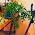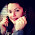### MATH 1280 Introduction to Statistics – Midterm Exam – part 6

One of Paul Cezanne’s paintings

Midterm Part 6 – questions 16, include its answer (completed-total questions are 16)

Some researchers collected data on the number of pairs of shoes that students own.  Five students owned seven pairs, two students owned no pairs, and various students owned between one and five pairs of shoes.

Please see the data in the frequency table:

 Shoes owned 0 1 2 3 4 5 7 Frequency 2 4 20 8 6 4 5

Card players, painted by Paul Cezanne.

16) What is the mean of the number of pairs of shoes that the students owned?
Select one:
a. 3.0 …> Correct
b. 3.1
c. 2.6
d. 2.9
e. 2.2

Total pair = 2(0) + 4(1) + 20(2) + 8(3) + 6(4) +4(5)+ 5(7) = 147
Total student: 49
Mean = 147/49 = 3

1.Oh math I have always hated math in school

1.Thank you to visit:
MATH 1280 Introduction to Statistics – Midterm Exam – part 6
-
One of Paul Cezanne’s paintings

Midterm Part 6 – questions 16, include its answer (completed-total questions are 16)

2.Great post dear :)

1.Thank you to visit:
MATH 1280 Introduction to Statistics – Midterm Exam – part 6
-
Some researchers collected data on the number of pairs of shoes that students own. Five students owned seven pairs, two students owned no pairs, and various students owned between one and five pairs of shoes.

Please see the data in the frequency table:

Shoes owned
0
1
2
3
4
5
7
Frequency
2
4
20
8
6
4
5

3.İnteresting post 😊 thanks for your sharing 😊

1.Thank you to visit:
MATH 1280 Introduction to Statistics – Midterm Exam – part 6
-
16) What is the mean of the number of pairs of shoes that the students owned?

Select one:
a. 3.0 …> Correct
b. 3.1
c. 2.6
d. 2.9
e. 2.2

Total pair = 2(0) + 4(1) + 20(2) + 8(3) + 6(4) +4(5)+ 5(7) = 147
Total student: 49
Mean = 147/49 = 3

4.For a while I tough I was in college again!!
xoxo

marisasclosetblog.com

1.Thank you to visit:
MATH 1280 Introduction to Statistics – Midterm Exam – part 6
-
One of Paul Cezanne’s paintings

Midterm Part 6 – questions 16, include its answer (completed-total questions are 16)

5.1.Thank you to visit:
MATH 1280 Introduction to Statistics – Midterm Exam – part 6
-
Some researchers collected data on the number of pairs of shoes that students own. Five students owned seven pairs, two students owned no pairs, and various students owned between one and five pairs of shoes.

Please see the data in the frequency table:
Shoes owned
0
1
2
3
4
5
7
Frequency
2
4
20
8
6
4
5

6.Oh very iteresting darling
This post It was something new to me
xx

1.Thank you to visit:
MATH 1280 Introduction to Statistics – Midterm Exam – part 6
-
Card players, painted by Paul Cezanne.

7.Enjoyed these wonderful paintings, the statistics is over my head.

1.Thank you to visit:
MATH 1280 Introduction to Statistics – Midterm Exam – part 6
-
16) What is the mean of the number of pairs of shoes that the students owned?

Select one:
a. 3.0 …> Correct
b. 3.1
c. 2.6
d. 2.9
e. 2.2

Total pair = 2(0) + 4(1) + 20(2) + 8(3) + 6(4) +4(5)+ 5(7) = 147
Total student: 49
Mean = 147/49 = 3

8.Very interesting post.
Greetings.

1.Thank you to visit:
MATH 1280 Introduction to Statistics – Midterm Exam – part 6
-
One of Paul Cezanne’s paintings

Midterm Part 6 – questions 16, include its answer (completed-total questions are 16)

9.Cezanne with two of my favorite things: fruit and card games. Pear season is nearly upon us.

1.I see.....yes, indeed

Thank you to visit:
MATH 1280 Introduction to Statistics – Midterm Exam – part 6
-
Some researchers collected data on the number of pairs of shoes that students own. Five students owned seven pairs, two students owned no pairs, and various students owned between one and five pairs of shoes.

Please see the data in the frequency table:
Shoes owned
0
1
2
3
4
5
7
Frequency
2
4
20
8
6
4
5

10.1.Thank you to visit:
MATH 1280 Introduction to Statistics – Midterm Exam – part 6
-
Card players, painted by Paul Cezanne.

11.I was never fond of statistics. I guess it's good that some people are, though.

1.Thank you to visit:
MATH 1280 Introduction to Statistics – Midterm Exam – part 6
-
16) What is the mean of the number of pairs of shoes that the students owned?
Select one:
a. 3.0 …> Correct
b. 3.1
c. 2.6
d. 2.9
e. 2.2

Total pair = 2(0) + 4(1) + 20(2) + 8(3) + 6(4) +4(5)+ 5(7) = 147
Total student: 49
Mean = 147/49 = 3

12.I guess it's good that some people are

1.Thank you to visit:
MATH 1280 Introduction to Statistics – Midterm Exam – part 6
-
One of Paul Cezanne’s paintings

Midterm Part 6 – questions 16, include its answer (completed-total questions are 16)

13.interesting information and images ☺
greetings
Lili

1.Thank you to visit:
MATH 1280 Introduction to Statistics – Midterm Exam – part 6
-
Some researchers collected data on the number of pairs of shoes that students own. Five students owned seven pairs, two students owned no pairs, and various students owned between one and five pairs of shoes.

Please see the data in the frequency table:
Shoes owned
0
1
2
3
4
5
7
Frequency
2
4
20
8
6
4

14.Well maybe if the students don't like mathematics, they can study Cezànne?

1.hope so....hehehe...lol...

Thank you to visit:
MATH 1280 Introduction to Statistics – Midterm Exam – part 6
-
Card players, painted by Paul Cezanne.

15.I love paintings and I don't love mathematics too :)

1.Thank you to visit:
MATH 1280 Introduction to Statistics – Midterm Exam – part 6
-
16) What is the mean of the number of pairs of shoes that the students owned?
Select one:
a. 3.0 …> Correct
b. 3.1
c. 2.6
d. 2.9
e. 2.2

Total pair = 2(0) + 4(1) + 20(2) + 8(3) + 6(4) +4(5)+ 5(7) = 147
Total student: 49
Mean = 147/49 = 3

16.Hi dear!
Great post, you have a very nice blog. <3
If you want a follow each other, write me on my blog. :)

1.Thank you to visit:
MATH 1280 Introduction to Statistics – Midterm Exam – part 6
-
One of Paul Cezanne’s paintings

Midterm Part 6 – questions 16, include its answer (completed-total questions are 16)

17.I love the pictures you pick to illustrate your posts - two great Cezanne paintings!

18.Thank you to visit:
MATH 1280 Introduction to Statistics – Midterm Exam – part 6
-
Some researchers collected data on the number of pairs of shoes that students own. Five students owned seven pairs, two students owned no pairs, and various students owned between one and five pairs of shoes.

Please see the data in the frequency table:
Shoes owned
0
1
2
3
4
5
7
Frequency
2
4
20
8
6
4
5